+
Application of z-Scores
Interpret Inferential Statistics
0
of 0 possible points

# Application of z-Scores

Author: Sophia Tutorial
##### Description:

Compute probability for normal models given a verbal description.

(more)

Sophia’s self-paced online courses are a great way to save time and money as you earn credits eligible for transfer to many different colleges and universities.*

No credit card required

37 Sophia partners guarantee credit transfer.

299 Institutions have accepted or given pre-approval for credit transfer.

* The American Council on Education's College Credit Recommendation Service (ACE Credit®) has evaluated and recommended college credit for 32 of Sophia’s online courses. Many different colleges and universities consider ACE CREDIT recommendations in determining the applicability to their course and degree programs.

Tutorial
what's covered
This lesson discusses the application of z-scores. By the end of the lesson, you should be able to use a z-score table to determine the probability of values associated with a real-world variable that is normally distributed. This lesson covers:
1. Application of z-Scores
2. Newborns
3. Tires

## 1. Application of z-Scores

With a normal distribution of a variable, the mean is the center of the distribution, while the standard deviation shows the level of variability that exists. When you know the value of a variable that is normally distributed, you can calculate the z-score by using the formula:

formula
z-Score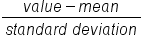When you are interested in determining the probability of a specific value occurring, you can use a z-table to look up areas for a calculated z-score to determine the area under the curve of any normal distribution. This can tell you the chance of a value occurring. It should be noted that not all z-score tables are the same.

term to know
z-Table
Table for looking up the area starting at z = 0 for a positive z-score

## 2. Newborns

Take a look at the weight of newborn babies. Suppose that the mean weight of newborns is 7.5 pounds and the standard deviation is 1.25 pounds. Say you’re interested in determining the probability that a newborn weighs less than 6 pounds. How do you do that?

Example: Newborns

Mean = 7.5 lbs
Standard Deviation = 1.25 lbs

The first thing you do is calculate the z-score. To figure out the z-score, take the difference between 6 and 7.5 to arrive at -1.5. When you divide -1.5 by the standard deviation of 1.25, you wind up with a z-score of -1.2.

Probability that a newborn weighs < 6 lbs
z-score = (6 - 7.5)/(1.25) = -1.20

When you’re looking at a z-table, the first step is to determine which row you want to look at. That’s going to be represented by the spot to the left of the decimal point and the first spot to the right of the decimal point, which is -1.2. The second step is to look at the digit that is two spots to the right of the decimal point, which is a 0. This determines the column you look at.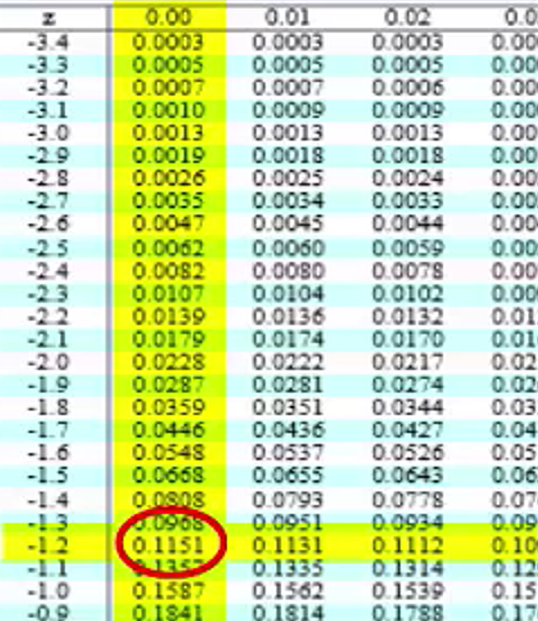The point at which both the row and the column intersect one another indicates the probability. The intersection in this scenario is 0.1151. That tells you that the probability associated with the baby weighing less than 6 pounds is 0.1151.

What if you’re interested in looking at the probability that a newborn might weight more than 10 pounds? You could calculate the z-score much the same way you did with the previous calculation:

Probability that a newborn weighs > 10 lbs z-score = (10 - 7.5)/(1.25) = 2.00

The same process is involved in looking at the z-table. Look at 2.0 and which row that entails. Since there are no numbers other than 0 to the right of the decimal point, you look at the very first column, which is the 0.00 column. Note, this table looks different from the one above. The z scores in the left column are negative instead of positive. For a full PDF on z-score tables, please see the attachment below this tutorial.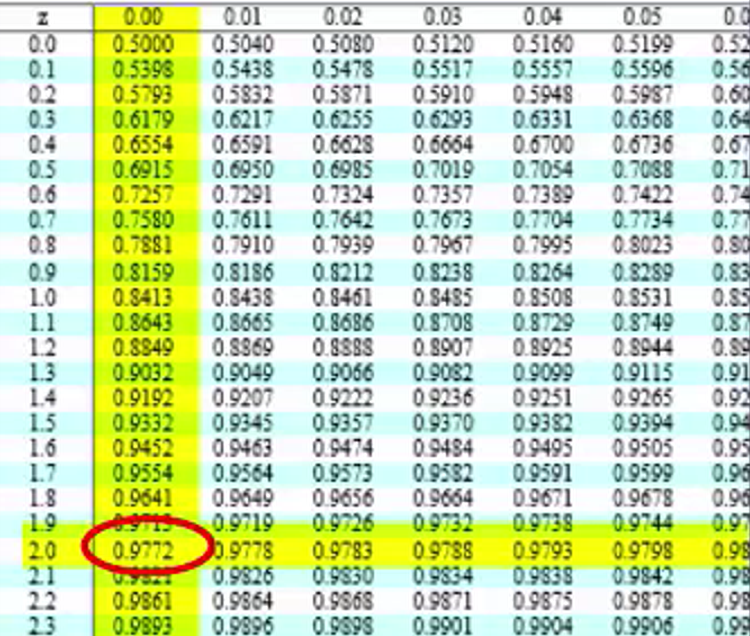You wind up with a probability of 0.9772. Remember, you are trying to determine the probability that a newborn weighs more than 10 pounds. The value given to you here is telling you the area underneath the curve to the left of +2 standard deviations, as you see on the graph right here: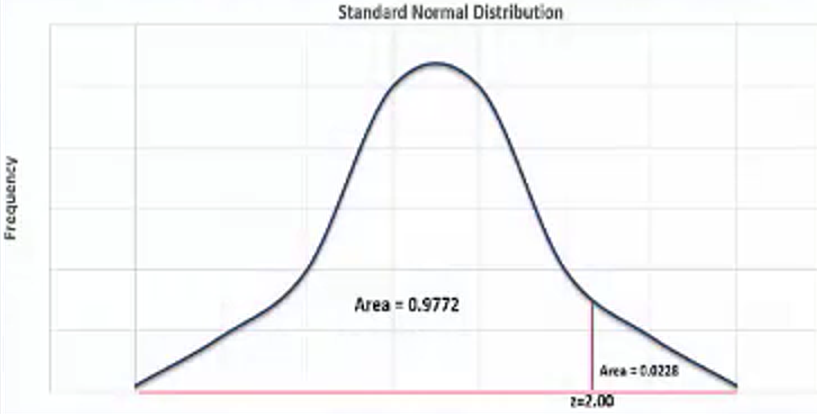You will need to subtract that value from 1 to get the area that’s on the right-hand side of that line. The probability that a newborn would be greater than 10 pounds would be 1 minus 0.9772. The probability is equal to 0.0228.

## 3. Tires

Take a look at something a little bit different: the average lifespan of car tires. Suppose that the population mean is 60,000 miles, and the standard deviation has been determined to be 8,000 miles. Imagine you’re interested in determining the probability that a tire lasts less than 45,500 miles.

What is the first step? You need to calculate the z-score.
z-score= (45,500 - 60,000)/(8,000) = -1.81

When you look that up on the z-table, remember that first you start with the -1.8. Since the second decimal point is a 0.01, you look at the second column.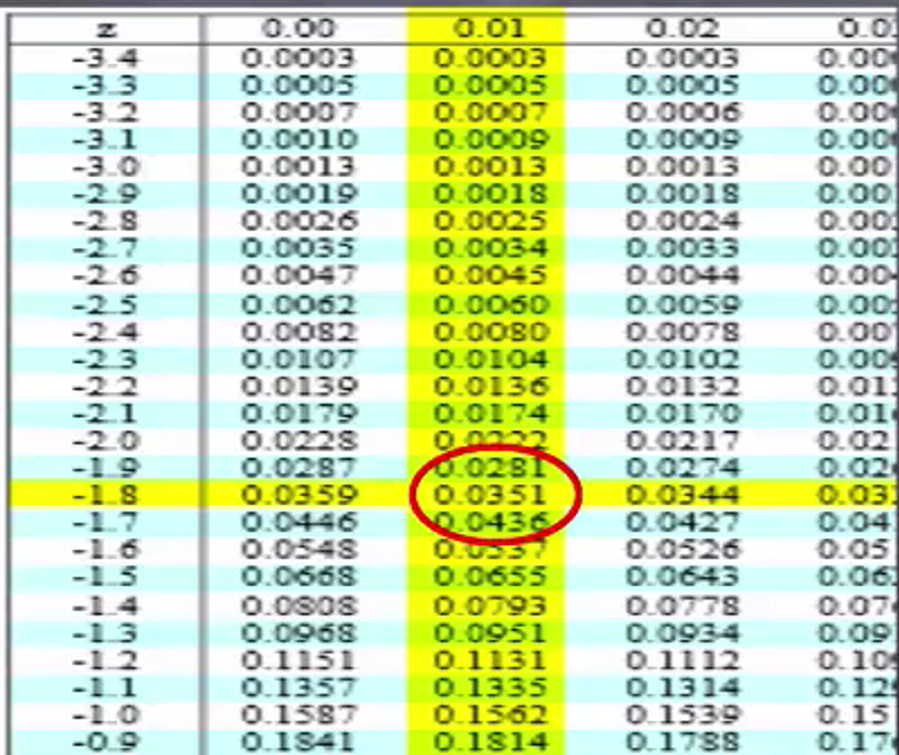The probability is equal to 0.0351.

What does this tell you? It tells you the probability that a tire would last less than 45,500 miles would be 0.0351.

What if you were interested in determining the probability that a tire would have a lifespan greater than 80,300 miles?

try it
Calculate the z-score. Did you get a score of 2.54? If not, take a look at the formula in use to check your math.
z-score = (80,300 - 60,000)/(8,000) = 2.54

Using the z-table, find the row that represents 2.5 and the column that represents 0.04: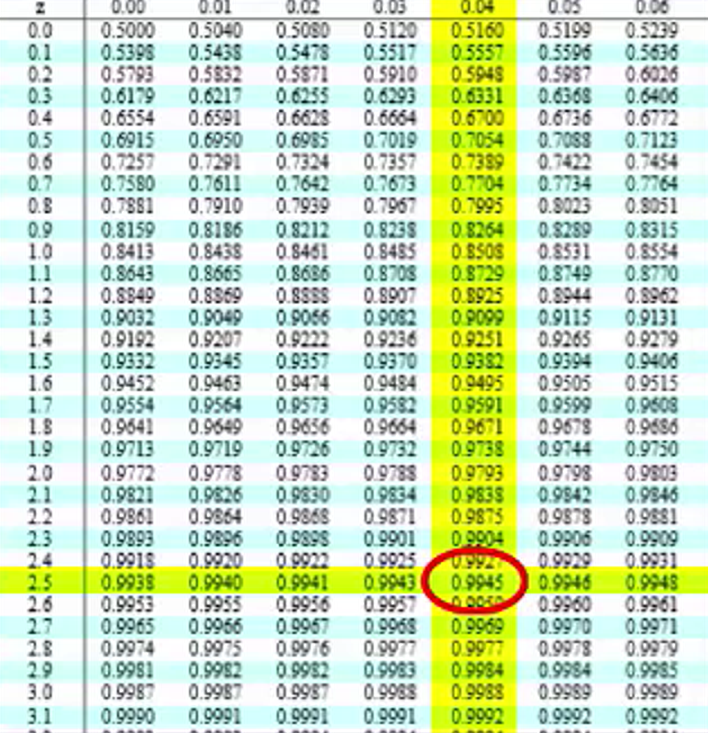The probability provided to us is 0.9945. That’s not the answer you’re looking for here. That would be the area underneath the normal distribution curve up to 2.54 standard deviations. You’re interested in the area to the right of that, and so you would need to subtract that value from 1. When you do, you get a probability 0.0055. In other words, it is very unlikely in this particular case that a tire would have a lifespan greater than 80,300 miles.

summary
Today you looked at the application of z-scores to determine the probability of values associated with a real-world variable that is normally distributed. You also went through a couple of examples of an assumed normally distributed variable, both the weight of newborns and the lifespan of tires. Using these examples, you saw how you could figure out, using a z-table, the probability of a value being less than or greater than a particular number.

Source: This work is adapted from Sophia author Dan Laub.

## Full z-score tables!

of
Terms to Know
Z-Table

Table for looking up the area starting at z=0 for a positive z-score.

Formulas to Know
Z-ScoreRating